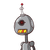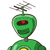# classifly the following into monominals binomal and trinomials (a) -6 (b) -5+×(c) 32× -4 (d) 6 ×2+5×3​

classifly the following into monominals binomal and trinomials (a) -6 (b) -5+×(c) 32× -4 (d) 6 ×2+5×3​

### 2 thoughts on “classifly the following into monominals binomal and trinomials (a) -6 (b) -5+×(c) 32× -4 (d) 6 ×2+5×3​”

1.(a) is monomial

b and c are binomial

d is polynomial

2.